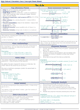How to Learn in 24 Hours?The Rapid Learning Movie

 Need Help? M-F: 9am-5pm(PST): Toll-Free: (877) RAPID-10 US Direct: (714) 692-2900 Int'l: 001-714-692-2900 24/7 Online Technical Support: The Rapid Support Center Secure Online Order:Need Proof? Testimonials by Our Users

 Rapid Learning Courses: MCAT in 24 Hours (2015-16) USMLE in 24 Hours (Boards) Chemistry in 24 Hours Biology in 24 Hours Physics in 24 Hours Mathematics in 24 Hours Psychology in 24 Hours SAT in 24 Hours ACT in 24 Hours AP in 24 Hours CLEP in 24 Hours DAT in 24 Hours (Dental) OAT in 24 Hours (Optometry) PCAT in 24 Hours (Pharmacy) Nursing Entrance Exams Certification in 24 Hours eBook - Survival Kits Audiobooks (MP3)

 Tell-A-Friend: Have friends taking science and math courses too? Tell them about our rapid learning system.Home »  Chemistry »  Highschool Chemistry

Counting Molecules: The Mole

 Topic Review on "Title": Atoms and molecules are so tiny that they need to be counted in very large quantities in order to result in a “workable” number.  The mole The mole (abbreviation: mol) is used to count particles.  1 mole of anything is 6.02 ´ 1023 of that thing.  Molar mass We cannot count out a mole of particles in the lab, therefore a connection between moles and mass is needed.  The molar mass is the mass (in grams) for 1 mole of the particle.  Molar mass (also often called Molecular mass, formula weight, etc.) is found by adding the atomic masses (multiplied by the number of that atom) for each atom in the molecule.  Molar mass can then be used in dimensional analysis conversions as the equality between grams and moles.  The molar mass value always goes with the “grams” in such conversions. Percent Composition “Percent” is part / whole ´ 100.  The “part” is the mass of an individual element while the “whole” is the mass of the whole molecule or sample.  These calculations can be done with lab data—grams of both the individual element and the whole sample—or with chemical formulas.  In the case of chemical formulas, the molar mass is used in the calculations. Empirical Formula The empirical formula is the lowest possible ratio of atoms in a molecule.  The ratio of atoms is the same as the ratio of moles of atoms.  The mass of each element is converted to moles and then all the moles are divided by the smallest to reach the lowest possible whole number ratio.  That ratio is used as the subscripts in writing the empirical formula. Molecular Formula The molecular formula is the actual ratio of atoms in a molecule.  The molar mass of the empirical formula is compared to the given molar mass of the molecular formula.  The comparison results in a factor that is used to multiply the subscripts of the empirical formula to reach the subscripts of the molecular formula.

Rapid Study Kit for "Title":
 Flash Movie Flash Game Flash Card Core Concept Tutorial Problem Solving Drill Review Cheat Sheet"Title" Tutorial Summary : Atoms and molecules are too small to be counted individually, so a counting unit of “mole” is used.  Molar masses are used to convert between mass and moles of atoms or molecules.  There are several ways to express the ratio of moles of atoms in a sample.  The tutorial introduces percent composition, empirical formula and molecular formula.

 Tutorial Features: Series Features: Concept map showing inter-connections of new concepts in this tutorial and those previously introduced. Definition slides introduce terms as they are needed. Visual representation of concepts Animated examples—worked out step by step A concise summary is given at the conclusion of the tutorial.

 "Title" Topic List: The Mole Mole relationships Molar Mass Calculating Using in mole conversions Percent Composition Empirical formulas Molecular formulas

See all 24 lessons in college chemistry, including concept tutorials, problem drills and cheat sheets:
Teach Yourself HighSchool Chemistry Visually in 24 Hours